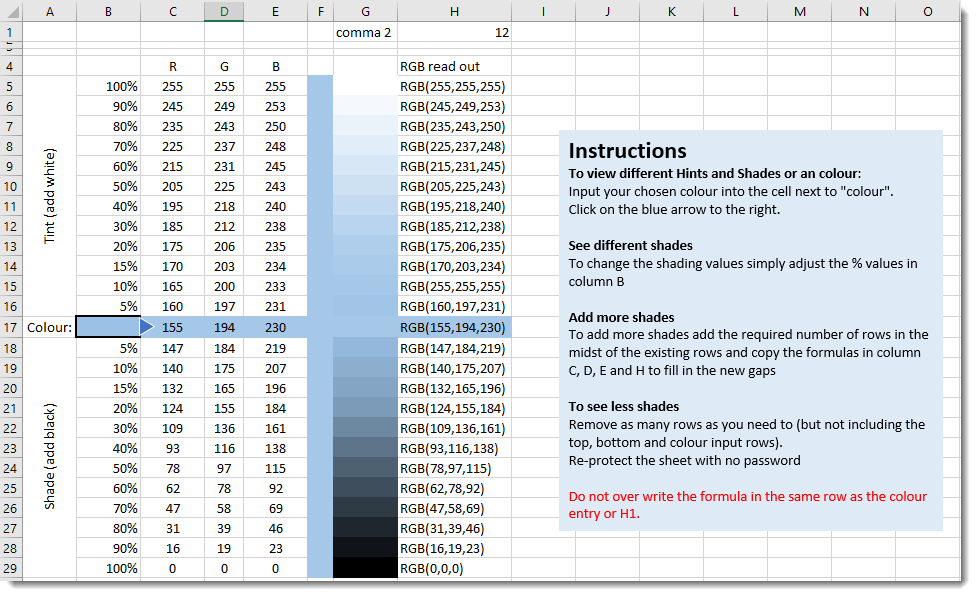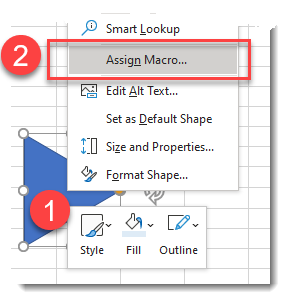Home > Blog

# Calculating Cell colour and changing Tints and Shades in Excel using VBA

When using SummaryPro you are able to select the colour that you want a shape to be in the Styles sheet.  This colour can then be applied to many different shapes in the final Plan on a Page.  To do this all you have to do is change the cell colour in the Excel engine and SummaryPro applies this in the Visio display.  To do this SummaryPro has to be able to identify the RGB value of the cell that you just changed.

This is done using a VBA module and I will use some of this in this example sheet.

However, when working with colours it is easy to change the shade of the colour by either making it lighter or darker.  Formally these two actions have different terms:

• Changing the Tint - adding more or less white to the colour
• Changing the Shade - adding more or less black to the colour..

We can programme VBA to be able to change the colour in this way once we have got the Red, Green, and Blue values for the cell.  To do this we only need to act on each of the R, G and B values in the following ways (shown against the R value in these instances)

• Change the tint: R = R +((255 - R) * % change)
• Change the shade: R = R * (1- % change)

Note that we have to round the values as R needs to be a whole number.   This is easy to go in the example sheet however in VBA it needs an additional trick which I will go into at the end.

In this blog I will walk you through the process of making the sheet shown below which illustrates the different Tints and Shades generated for any given colour.To build this sheet first set up the columns A and B then insert the following formulas:

• H1 =FIND(",",H17,FIND(",",H17)+1)
• C5 =ROUND(C\$17+((255-C\$17)*\$B5),0)
• D5 =ROUND(D\$17+((255-D\$17)*\$B5),0)
• E5 =ROUND(E\$17+((255-E\$17)*\$B5),0)
• H5 ="RGB("&C5&","&D5&","&E5&")"

Copy C5:H5 down to row 16

• C17 =MID(H17,5,FIND(",",H17)-5)*1
• D17 =MID(H17,FIND(",",H17)+1,H1-FIND(",",H17)-1)*1
• E17 =MID(H17,H1+1,LEN(H17)-H1-1)*1
• C18 =ROUND(C\$17*(1-\$B18),0)
• D18 =ROUND(D\$17*(1-\$B18),0)
• E18 =ROUND(E\$17*(1-\$B18),0)
• H18 ="RGB("&C18&","&D18&","&E18&")"

Copy C18:H18 down to Row 29

Make B17 the colour of your choice and marge F5:F29

Now we need to add some VBA.

• Press the <ALT> key and F11 at the same time to bring up the VAB editor
• Press Insert / Module to insert a blank coding space in your sheet.
• Copy the code below (below but not including the START and END CODE lines) in to this blank space.

---START CODE---

Option Explicit

Sub get_colour()
Application.ScreenUpdating = False
Application.Calculation = xlCalculationManual

On Error GoTo ErrHandle:
Dim target As Range 'any of the pick columns, will be re-set each time
Dim wb As Workbook
Dim wsc As Worksheet
Dim sInteriorColor As String
Dim r As Integer
Dim G As Integer
Dim B As Integer
Dim i As Integer
Dim ColALast As Integer
Dim ColhLast As Integer
Dim n As Integer
Dim InputRow As Integer

Set wb = ActiveWorkbook
Set wsc = wb.Sheets("sheet1")
'identify where the colour is being entered as we can add rows.

ColALast = wsc.Range("a65536").End(xlUp).Row
For n = 1 To ColALast
If wsc.Range("A" & n) = "Colour:" Then InputRow = n
Next n

' aquire the RGB string of the target cell (B15)

Set target = wsc.Range("b" & InputRow)
If target.Interior.ColorIndex = -4142 Then 'for some reason -4142 appears to be blank rather than 0 as expected
sInteriorColor = "RGB(255,255,255)"
Else
sInteriorColor = Hex(target.Interior.Color)
sInteriorColor = "000000" & sInteriorColor
sInteriorColor = Right(sInteriorColor, 6)
sInteriorColor = "RGB(" & CInt("&H" & Right(sInteriorColor, 2)) & _
"," & CInt("&H" & Mid(sInteriorColor, 3, 2)) & _
"," & CInt("&H" & Left(sInteriorColor, 2)) & ")"
End If
'display the RGB value of the target cell
wsc.Range("h" & InputRow).Value = sInteriorColor
'switch on calculation to allow the cells to change RGB values in columns C:E

ColhLast = wsc.Range("h65536").End(xlUp).Row

Application.Calculation = xlCalculationAutomatic

'Set the interior colour for each of the shade and tint outcomes
For i = 5 To ColhLast
r = wsc.Range("c" & i)
G = wsc.Range("d" & i)
B = wsc.Range("e" & i)
wsc.Range("g" & i).Interior.Color = RGB(r, G, B)
Next i

'set the colour of the merged cell in F:F to match that of the target cell
r = wsc.Range("c15")
G = wsc.Range("d15")
B = wsc.Range("e15")
wsc.Range("f5").Interior.Color = RGB(r, G, B)
wsc.Range("C" & InputRow & ":h" & InputRow).Interior.Color = RGB(r, G, B)

Application.ScreenUpdating = True

Exit Sub
ErrHandle:
If Err.Number > 0 Then
Application.Calculation = xlCalculationAutomatic
Application.ScreenUpdating = True
End If
End Sub

---END CODE---

If you hit the play button on the menu the code will run however it is much easier to trigger it from a button on the sheet.  To do this simply insert any shape (in my example I chose to use a triangle positioned by inserted colour and then right mouse on this drawing object and chose to assign the macro "get_colour".I mentioned that if you move the ROUND() function into VBA then you will need to use a trick as when VBA rounds it does so using a form or rounding called "Banker's rounding". This is best explained in this blog post on TechNet which can allow you to use StandardRound() instead of the included Round(). Note this only applies if you're doing rounding in VBA.Miles Goodchild has been a Program and Programme Planner, PMO lead and Project Manger since 1998.  He enjoys using MS Office, especially Project, Excel and Visio to make life easier and simpler.  In the course of this he created SummaryPro.  In this blog he shares some of the tips and techniques he has learnt over the years in the hope that they will be useful to you.